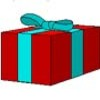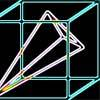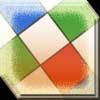#### You may also likeA box of size a cm by b cm by c cm is to be wrapped with a square piece of wrapping paper. Without cutting the paper what is the smallest square this can be?### Four Points on a Cube

What is the surface area of the tetrahedron with one vertex at O the vertex of a unit cube and the other vertices at the centres of the faces of the cube not containing O?### Instant Insanity

We have a set of four very innocent-looking cubes - each face coloured red, blue, green or white - and they have to be arranged in a row so that all of the four colours appear on each of the four long sides of the resulting cuboid.

# Plane to See

##### Age 16 to 18 Challenge Level:

Why do this problem?
It provides good eperience of visualisation in 3D and using ideas of similarity and scaling in 2D and 3D. They will need the formula for the volume of a tetrahedron.

It is not necesary to use 3D coordinates but they can be useful.

Possible approach
Emphasise the value of trying to visualise the plane cutting the cube. Suggesthe students close there eyes and try to visualise where the plane cuts the vertical line through $A$.

Suggest students look for similar triangles and similar 3D shapes.

Key question
Where does the line $EH$ cut the plane $PDQ$? Call this point $R$.

Where do the lines $PQ$ and $DR$ meet?